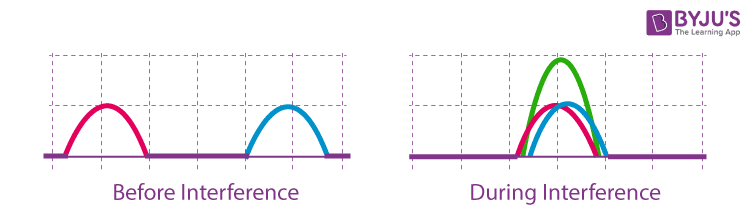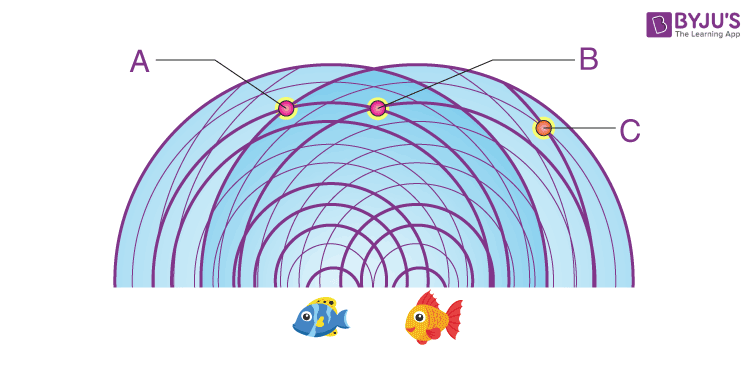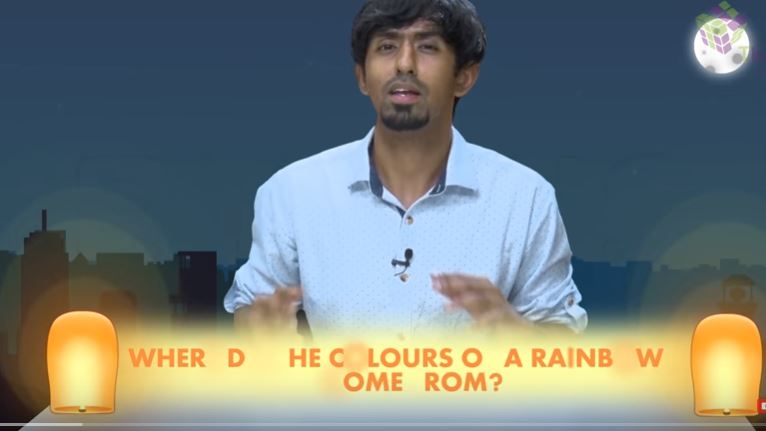# Interference of Waves

Have you ever wondered what happens when two waves travelling in the same medium meet? Will the two waves bounce off each other, or do they pass through each other? Or, will the meeting of two waves affect the appearance of the medium? These questions about the meeting of waves pertain to the topic of wave interference. In this article, we will discuss wave interference definition and its types in detail.

## What is Interference?

Interference is what happens when two or more waves meet each other. Depending on the overlapping waves’ alignment of peaks and troughs, they might add up, or they can partially or entirely cancel each other. As per the interference definition, it is defined as

, The phenomenon in which two or more waves superpose to form a resultant wave of greater, lower or the same amplitude.

The interference of waves results in the medium taking on a shape resulting from the net effect of the two individual waves. To better understand, let us consider the example of two pulses of the same amplitude travelling in different directions in the same medium. Let us consider each displaced upward by 1 unit at its crest and has the shape of a sine wave. As these sine pulses move towards each other, there will be a moment in time when they are completely overlapped. At this point, the shape of the medium would be an upward displaced sine wave with an amplitude of 2 units. After understanding the interference definition, let us learn the derivation of wave interference.

### DerivationThe figure illustrates the before and during interference snapshots of the medium of two pulses. The individual sine pulses are drawn in pink and blue, and the resulting wave is green in colour.
The principle of superposition of waves states that when two or more waves of the same type are incident on the same point, the resultant amplitude at that point is equal to the vector sum of the amplitudes of the individual waves. If the crest of a wave meets the crest of another wave of the same frequency at the same point, then the resultant amplitude is the sum of individual amplitudes – this is known as constructive interference. Similarly, suppose a wave’s crest meets another wave’s trough. In that case, the resultant amplitude is equal to the difference in the individual amplitudes – this is known as destructive interference. The formula for the sum of two waves can be derived as follows:

The amplitude of a sinusoidal wave travelling to the right along the x-axis is given by,

$$\begin{array}{l}W_1(x,t)=A\cos (kx-\omega t)\end{array}$$

Where A is the peak amplitude, k = 2π/λ is the wavenumber and ω = 2πf is the angular frequency of the wave.

Consider another wave of the same frequency and amplitude but with a different phase travelling to the right.

$$\begin{array}{l}W_2(x,t)=A\cos (kx-\omega t+\phi )\end{array}$$

where φ is the phase difference between the waves in radians

The two waves superimpose and add; the equation gives the resultant wave,

$$\begin{array}{l}W_1+W_2=A[\cos (kx-\omega t)+\cos (kx-\omega t+\phi)]\end{array}$$
(1)

The equation gives the sum of two cosines,

$$\begin{array}{l}\cos a+\cos b=2\cos (\frac{a-b}{2})\cos (\frac{a+b}{2})\end{array}$$

Solving equation (1) using the formula, we get

$$\begin{array}{l}W_1+W_2=2A\cos \frac{\phi }{2}\cos (kx-\omega t+\frac{\phi }{2})\end{array}$$

Constructive Interference: When the phase difference is an even multiple of π (φ = ….., –4π, –2π, 0, 2π, 4π,……), then cos φ/2 =1, so the sum of the two waves is a wave with twice the amplitude.

$$\begin{array}{l}W_1+W_2=2A\cos (kx-\omega t)\end{array}$$

Destructive Interference: When the phase difference is an odd multiple of π (φ =….., –3π, –π, 0, π, 3π, 5π,……), then cos φ/2 = 0, so the sum of the two waves will be zero.

$$\begin{array}{l}W_1+W_2= 0\end{array}$$

Two fishes create a series of circular waves by swimming in the water. The waves undergo interference and create the pattern as represented in the diagram. The thick lines represent crests, and the thin lines represent troughs. Several positions in the diagram are labelled with a letter. Categorize the labelled position as being either constructive or destructive interference.Constructive Interference: A and B

Destructive Interference: CQ1

### State the interference definition.

As per the interference definition, it is defined as the phenomenon in which two waves superpose to form the resultant wave of the lower, higher or same amplitude.

Q2

### What are the types of light interference?

The following are the types of light interference:

• Constructive interference
• Destructive interference
Q3

TRUE.
Q4

### Do sound waves undergo interference?

Yes, sound waves can undergo interference.
Q5

### State the principle of superposition of waves?

The principle of superposition of waves states that when two or more waves of the same type are incident on the same point, the resultant amplitude at that point is equal to the vector sum of the amplitudes of the individual waves.

Test your knowledge on Interference Of Waves